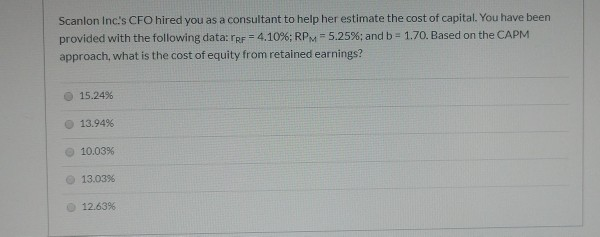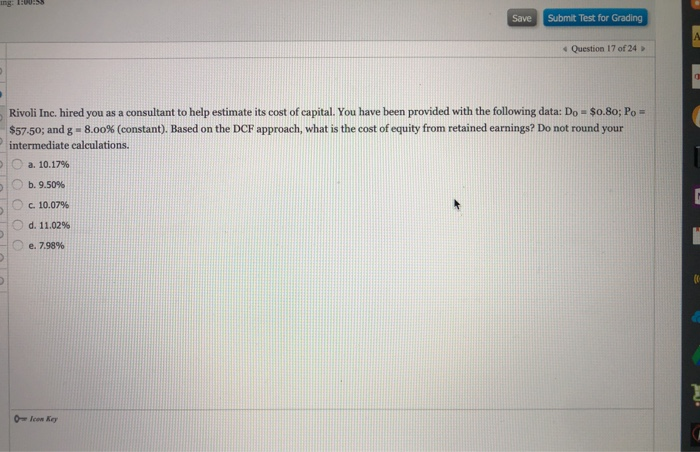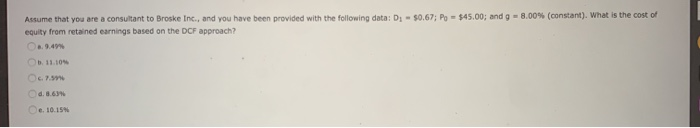Question

# Assume that GBIST Inc. hired you as a consultant to help estimate its cost of capital....

Assume that GBIST Inc. hired you as a consultant to help estimate its cost of capital. You have obtained the following data: D0 = \$1.20; P0 = \$1,200.00; and g = 6.00% (constant). Based on the DCF approach, what is the cost of equity from retained earnings?

Cost of equity=(D1/Current price)+Growth rate

=[(1.2*1.06)/1200]+0.06

which is equal to

=6.106%

#### Earn Coins

Coins can be redeemed for fabulous gifts.

Similar Homework Help Questions
• ### Rivoli Inc. hired you as a consultant to help estimate its cost of common equity. You...

Rivoli Inc. hired you as a consultant to help estimate its cost of common equity. You have been provided with the following data: D0 = \$0.80; P0 = \$22.50; and g = 8.00% (constant). Based on the DCF approach, what is the cost of common from retained earnings? Answer 10.69% 11.25% 11.84% 12.43% 13.05%

• ### Teall Development Company hired you as a consultant to help them estimate its cost of capital....

Teall Development Company hired you as a consultant to help them estimate its cost of capital. You have been provided with the following data: D1 = \$1.45; P0 = \$26.00; and g = 6.50% (constant). Based on the DCF approach, what is the cost of equity from retained earnings? Group of answer choices 12.68% 11.84% 12.08% 10.63% 9.78%

• ### Rivoli Inc. hired you as a consultant to help estimate its cost of capital. You have...

Rivoli Inc. hired you as a consultant to help estimate its cost of capital. You have been provided with the following data: D0 = \$0.80; P0 = \$77.50; and g = 8.00% (constant). Based on the DCF approach, what is the cost of equity from retained earnings? Group of answer choices 8.66% 7.20% 10.12% 9.11% 10.30% Malholtra Inc. is considering a project that has the following cash flow and WACC data. What is the project's MIRR? Note that a project's...

• ### Heino Inc., hired you as a consultant to help them estimate their cost of capital.  You have...

Heino Inc., hired you as a consultant to help them estimate their cost of capital.  You have been provided with the following data:  risk-free rate = 5%; market risk premium = 6.0%, and beta = 1.05.  Based on the CAPM approach, what is the cost of equity from retained earnings given a flotation cost of 10%? 10.50% / 10.71% / 10.88% / 11.30% / 11.60%

• ### Landmark, Inc. hired you as a consultant to help them estimate its cost of capital. You...

Landmark, Inc. hired you as a consultant to help them estimate its cost of capital. You have been provided with the following data: its most recently paid dividend is \$1.25; its current market price is \$31.50; its ROE = 12% and its dividend payout ratio is 40%. New common stock will have an 8% flotation cost. Based on the DCF approach and the Retention Growth Model, what is the cost of equity from new common stock? Enter your answer rounded...

• ### Assume that you are a consultant to Broske Inc., and you have been provided with the...

Assume that you are a consultant to Broske Inc., and you have been provided with the following data: D1 = \$2.10; P0 = \$49.50; and g = 6.00% (constant). What is the cost of equity from retained earnings based on the DCF approach? Enter your answer rounded to two decimal places. Do not enter % in the answer box. For example, if your answer is 0.12345 or 12.345% then enter as 12.35 in the answer box.

• ### Scanlon Inc.'s CFO hired you as a consultant to help her estimate the cost of capital....Scanlon Inc.'s CFO hired you as a consultant to help her estimate the cost of capital. You have been provided with the following data:TRF4.10%; RPM=5.25%; and b = 1.70. Based on the CAPM approach, what is the cost of equity from retained earnings? 15.24% 13.94% 10.03% 13.03% 12.63%

• ### You have been hired by the CFO of Lugones Industries to help estimate its cost of...

You have been hired by the CFO of Lugones Industries to help estimate its cost of common equity. You have obtained the following data: (1) rd = yield on the firm's bonds = 7.00% and the risk premium over its own debt cost = 4.00%. (2) rRF = 5.00%, RPM = 6.00%, and b = 1.25. (3) D1 = \$1.20, P0 = \$35.00, and g = 8.00% (constant). You were asked to estimate the cost of common based on the...

• ### ing: 1:00:55 Save Submit Test for Grading Question 17 of 24 Rivoli Inc. hired you as...ing: 1:00:55 Save Submit Test for Grading Question 17 of 24 Rivoli Inc. hired you as a consultant to help estimate its cost of capital. You have been provided with the following data: Do - \$0.80; P - \$57.50; and g - 8.00% (constant). Based on the DCF approach, what is the cost of equity from retained earnings? Do not round your intermediate calculations. a. 10.17% 5. b. 9.50% Soc. 10.07% O d. 11.02% e. 7.98% . 0 Icow Key

• ### Assume that you are a consultant to Broske Inc., and you have been provided with the...Assume that you are a consultant to Broske Inc., and you have been provided with the following data: D: - \$0.67; P - \$45.00; and g = 8.00% (constant). What is the cost of equity from retained earnings based on the DCF approach? 9.49 11.10 Oceni 1. Lupiel , SELUUN.10, Flue 50 Which of the following is NOT a capital component when calculating the weighted average cost of capital (WACC) for use in capital budgeting? a. Common stock. b. Preferred...